# CS224n笔记8 RNN和语言模型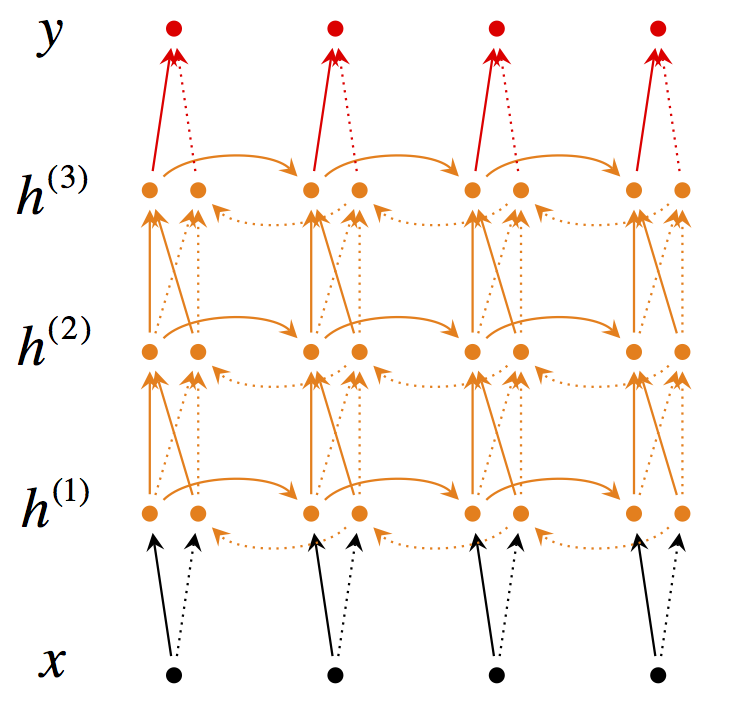这次课推导RNN，介绍各种训练技巧和拓展变种。梯度消失的推导很详细，用Python演示很直观，也给出了用裁剪防止梯度爆炸的直观解释。笔记里还补充了用于机器翻译时的5项改进。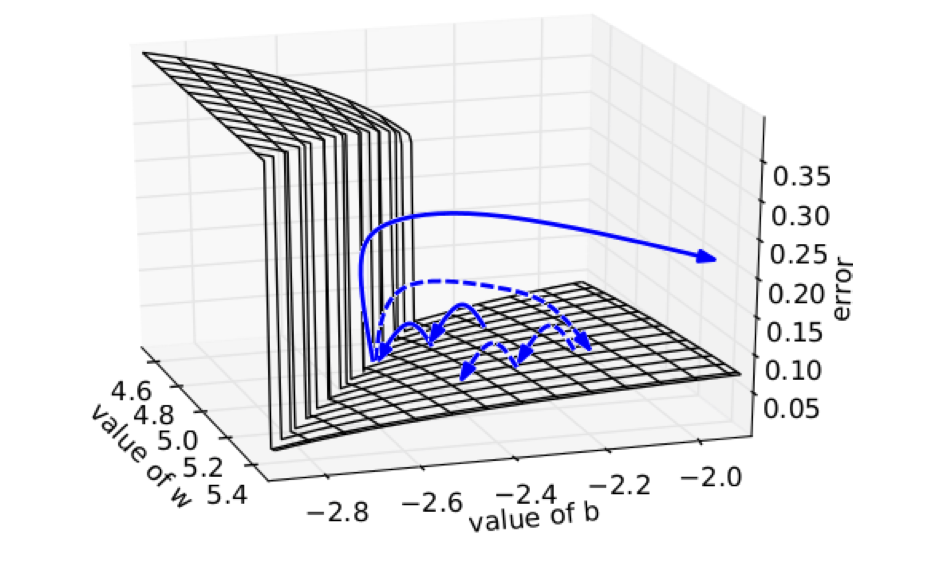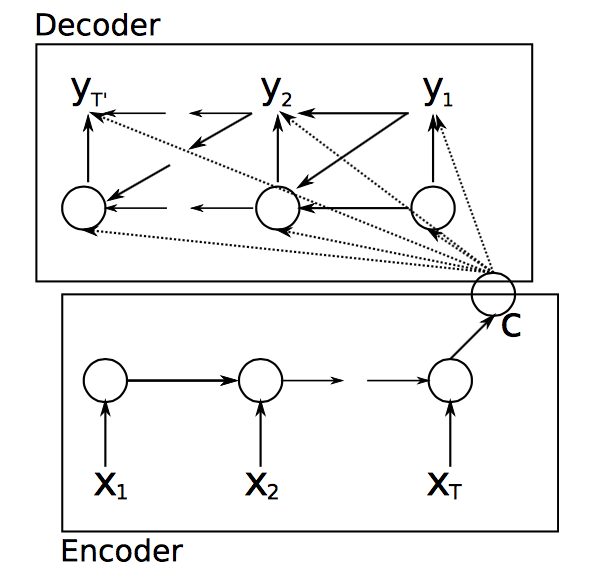## 语言模型

### 传统语言模型

$$\begin{equation} P(w_1,…,w_m) = \prod_{i=1}^{i=m} P(w_{i} | w_1, …, w_{i-1}) \approx \prod_{i=1}^{i=m} P(w_{i} | w_{i-n}, …, w_{i-1}) \label{eqn:nat_model} \end{equation}$$

\begin{align} p(w_2 | w_1) &= \dfrac {count(w_1,w_2)}{count(w_1)} \\ p(w_3 | w_1, w_2) &= \dfrac {count(w_1,w_2,w_3)}{count(w_1, w_2)} \end{align}

Bengio et al提出了第一个大规模深度学习自然语言处理模型，只不过是用前$n$个单词的词向量来做同样的事情（上文建模）而已，其网络结构如下：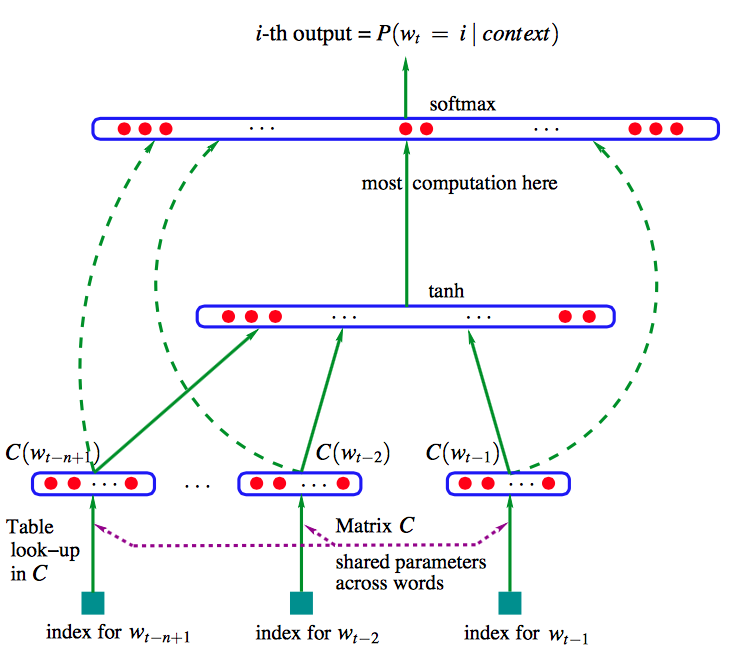$$\begin{equation} \hat{y} = softmax (W^{(2)} tanh(W^{(1)}x+b^{(1)})+W^{(3)}x+b^{(3)}) \label{eqn:bengio_eqn} \end{equation}$$

## Recurrent Neural Networks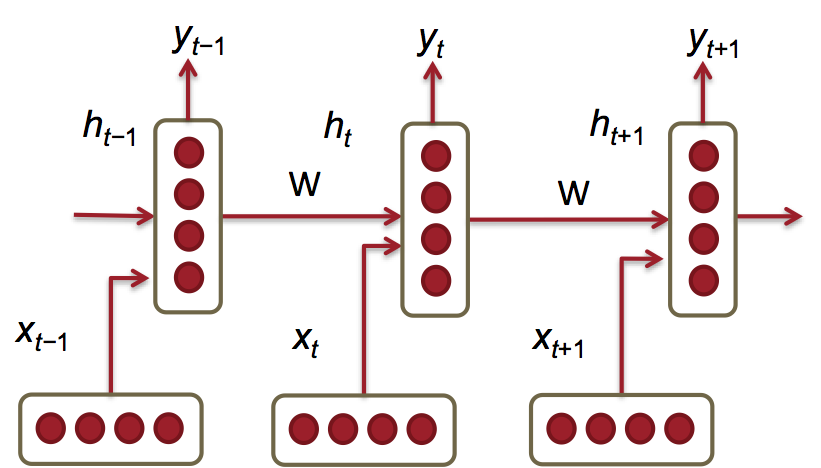$$h_t = \sigma(W^{(hh)} h_{t-1} + W^{(hx)} x_{t})$$

$x_{t} \in \mathbb{R}^{d}$：是时间$t$时输入的单词的词向量。

$W^{hx} \in \mathbb{R}^{D_h \times d}$：用来condition输入词向量$x_t$的的权值矩阵。

$W^{hh} \in \mathbb{R}^{D_h \times D_h}$：用来condition前一个时间节点隐藏层特征表示$h_{t-1}$的权值矩阵。

$h_{t-1} \in \mathbb{R}^{D_h}$: 前一个时间点 $t-1$ 的非线性激活函数的输出， $h_0 \in \mathbb{R}^{D_h}$ 是时间点 $t = 0$ 时的隐藏层初始状态。

$\sigma ()$: 非线性激活函数(sigmoid)

$\hat{y}_t = softmax (W^{(S)}h_t)$：在时刻$t$时输出的整个词表 $|V|$ 上的概率分布，$\hat{y}_t$是给定上文$h_{t-1}$和最近的单词 $x^{(t)}$预测的下一个单词。其中$W^{(S)} \in \mathbb{R}^{|V| \times D_h}$ ， $\hat{y} \in \mathbb{R}^{|V|}$。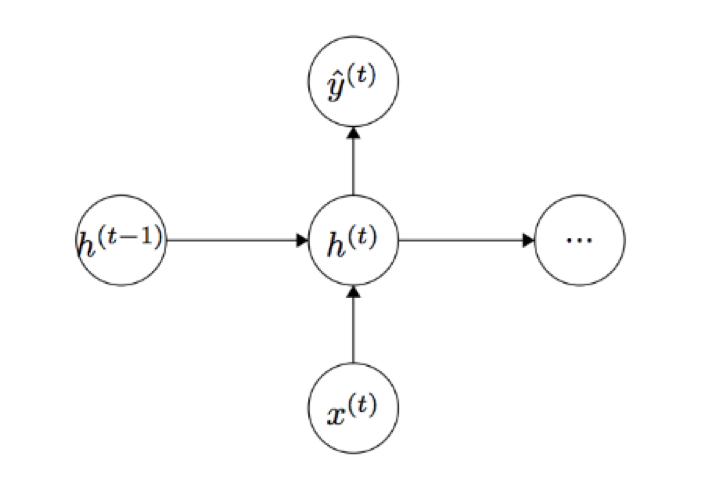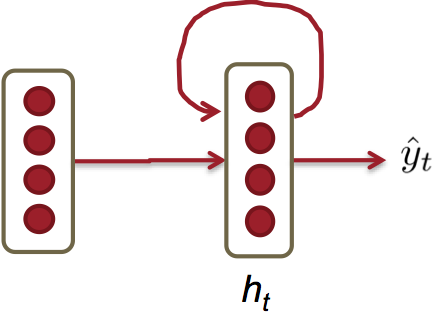### 损失函数

$$\begin{equation} J^{(t)}(\theta) = - \sum_{j=1}^{|V|} y_{t,j} \times log (\hat{y}_{t,j}) \label {eqn:rnn_loss} \end{equation}$$

$$\begin{equation} J = \dfrac{1}{T} \sum_{t=1}^{T} J^{(t)}(\theta) = - \dfrac{1}{T} \sum_{t=1}^{T} \sum_{j=1}^{|V|} y_{t,j} \times log (\hat{y}_{t,j}) \label {eqn:rnn_loss_T} \end{equation}$$

$$\begin{equation} Perplexity = 2^{J} \label{eqn:perplexity} \end{equation}$$

### 训练RNN很难

"Jane walked into the room. John walked in too. Jane said hi to ___"

"Jane walked into the room. John walked in too. It was late in the day, and everyone was walking home after a long day at work. Jane said hi to ___"

$$\begin{equation} \dfrac{\partial E}{\partial W} = \sum_{t=1}^{T}\dfrac{\partial E_t}{\partial W} \label{eqn:bp_rnn_error} \end{equation}$$

$$\begin{equation} \dfrac{\partial E_t}{\partial W} = \sum_{k=1}^{t} \dfrac{\partial E_t}{\partial y_t} \dfrac{\partial y_t}{\partial h_t} \dfrac{\partial h_t}{\partial h_k} \dfrac{\partial h_k}{\partial W} \label{eqn:bp_rnn_chain} \end{equation}$$

$$\begin{equation} \dfrac{\partial h_t}{\partial h_k} = \prod_{j=k+1}^{t}\dfrac{\partial h_j}{\partial h_{j-1}} = \prod_{j=k+1}^{t}W^T \times diag [f'(j_{j-1})] \label{eqn:bp_rnn_k} \end{equation}$$

$$\begin{equation} \dfrac{\partial h_j}{\partial h_{j-1}} = {[\dfrac{\partial h_{j}}{\partial h_{j-1,1}} ... \dfrac{\partial h_{j}}{\partial h_{j-1,D_n}}]} = \begin{bmatrix} \dfrac{\partial h_{j,1}}{\partial h_{j-1,1}} & . & . & . & \dfrac{\partial h_{j,1}}{\partial h_{j-1,D_n}} \\ . & . & & & . \\ . & & . & & . \\ . & & & . & . \\ \dfrac{\partial h_{j,D_n}}{\partial h_{j-1,1}} & . & . & . & \dfrac{\partial h_{j,D_n}}{\partial h_{j-1,D_n}} \\ \end{bmatrix} \label{eqn:bp_rnn_jaocb} \end{equation}$$

$$\begin{equation} \dfrac{\partial E}{\partial W} = \sum_{t=1}^{T}\sum_{k=1}^{t} \dfrac{\partial E_t}{\partial y_t} \dfrac{\partial y_t}{\partial h_t} (\prod_{j=k+1}^{t}\dfrac{\partial h_j}{\partial h_{j-1}}) \dfrac{\partial h_k}{\partial W} \end{equation}$$

$$\begin {equation} \parallel \dfrac{\partial h_j}{\partial h_{j-1}} \parallel \leq \parallel W^T\parallel \parallel diag [f'(h_{j-1})]\parallel \leq \beta_W \beta_h \label{eqn:bp_rnn_k_norm} \end {equation}$$

$$\begin {equation} \parallel \dfrac{\partial h_t}{\partial h_k} \parallel = \parallel \prod_{j=k+1}^{t} \dfrac{\partial h_j}{\partial h_{j-1}}\parallel \leq (\beta_W \beta_h)^{t-k} \label{eqn:bp_rnn_k_norm_total} \end {equation}$$

### 梯度消失实例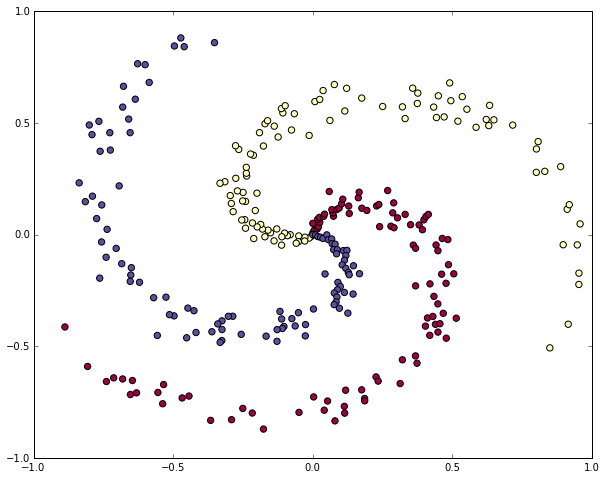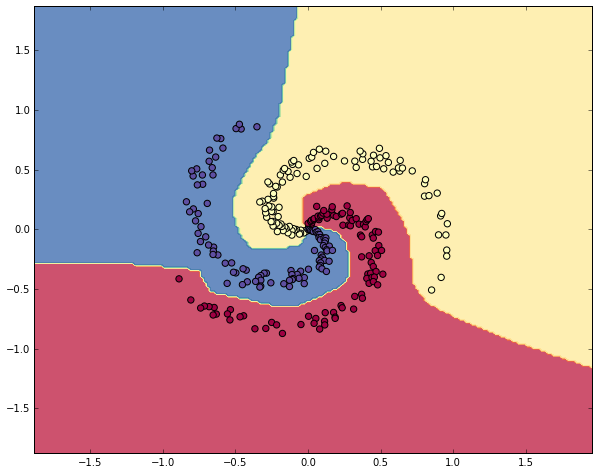sigmoid激活函数下：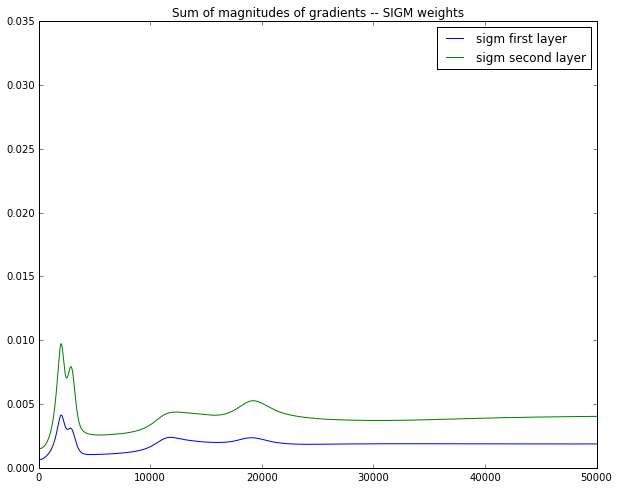ReLU激活函数下：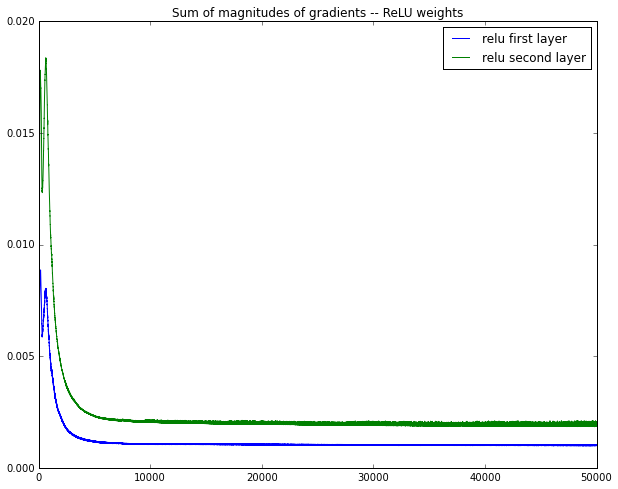### 防止梯度爆炸### 减缓梯度消失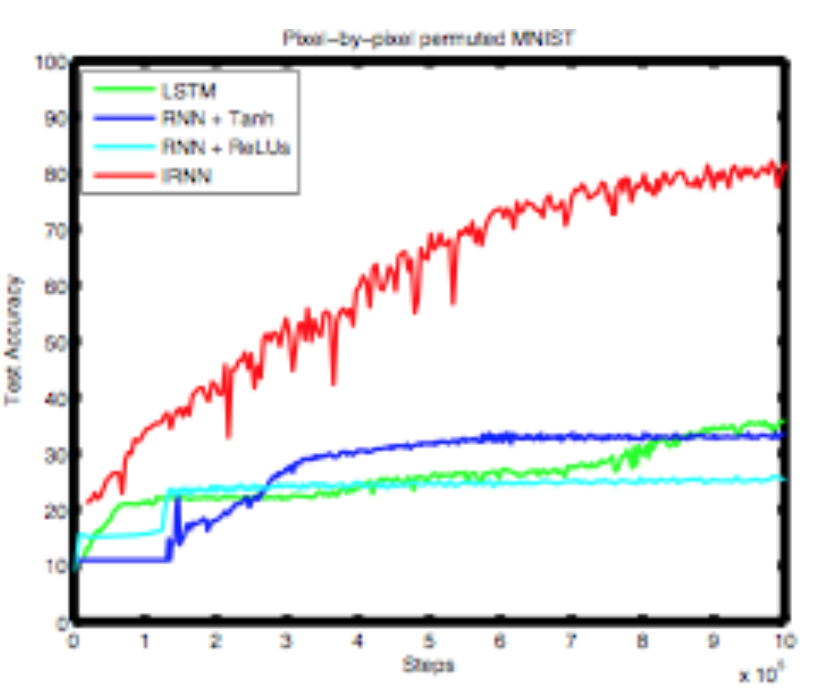### 困惑度结果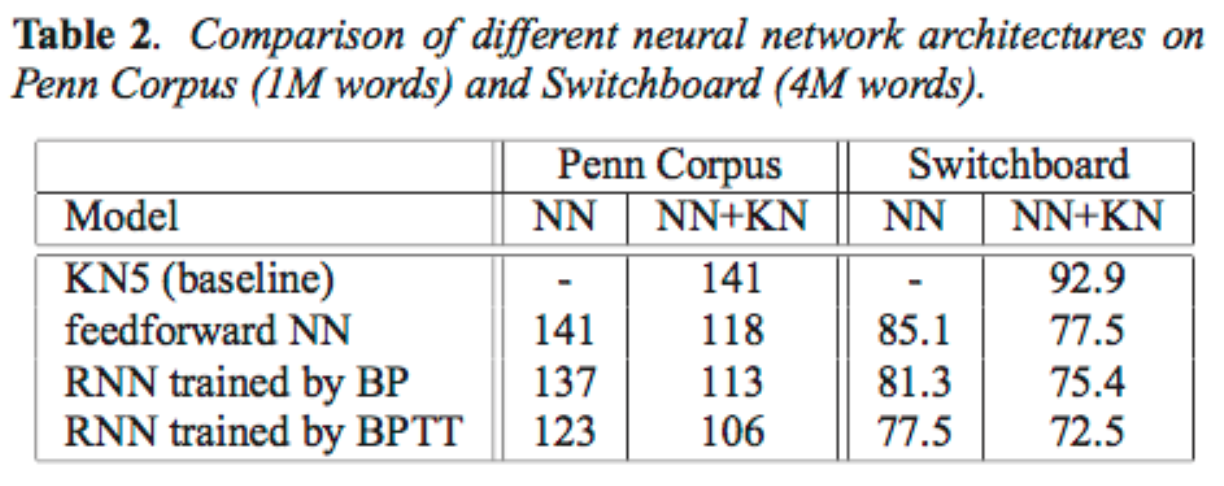### 问题：softmax太大了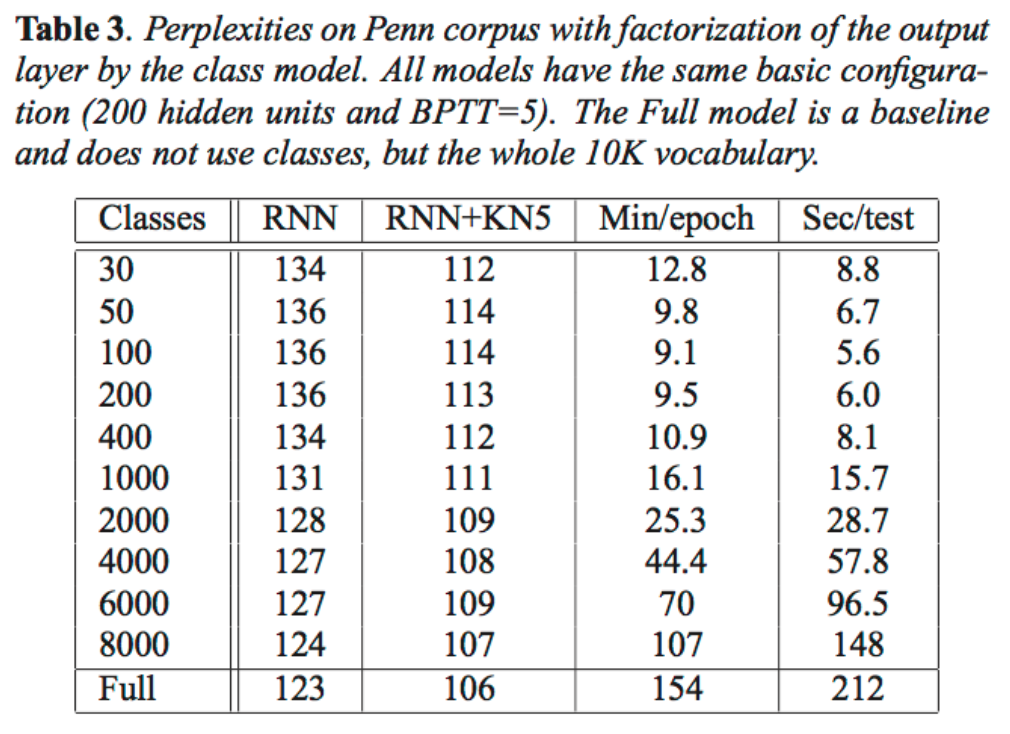## 序列模型的应用

DSE：直接主观描述（明确表达观点等）

ESE：间接主观描述（间接地表达情感等）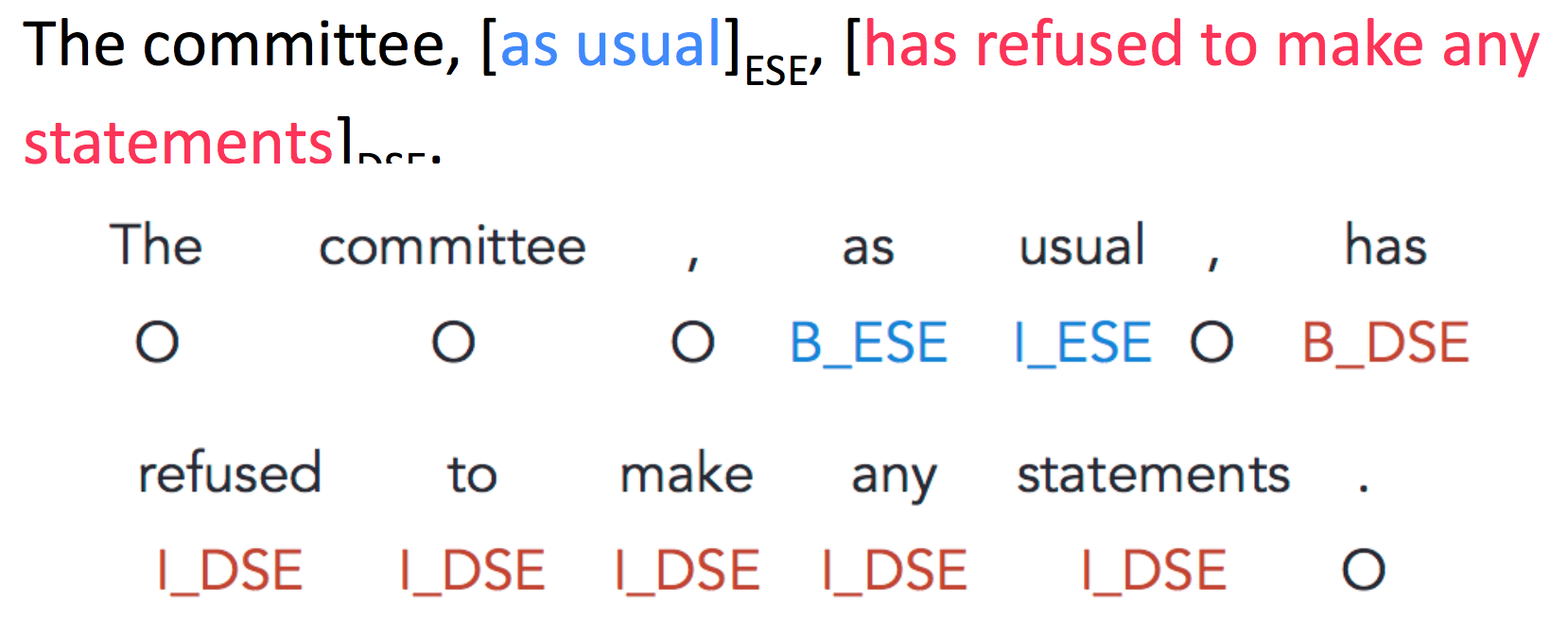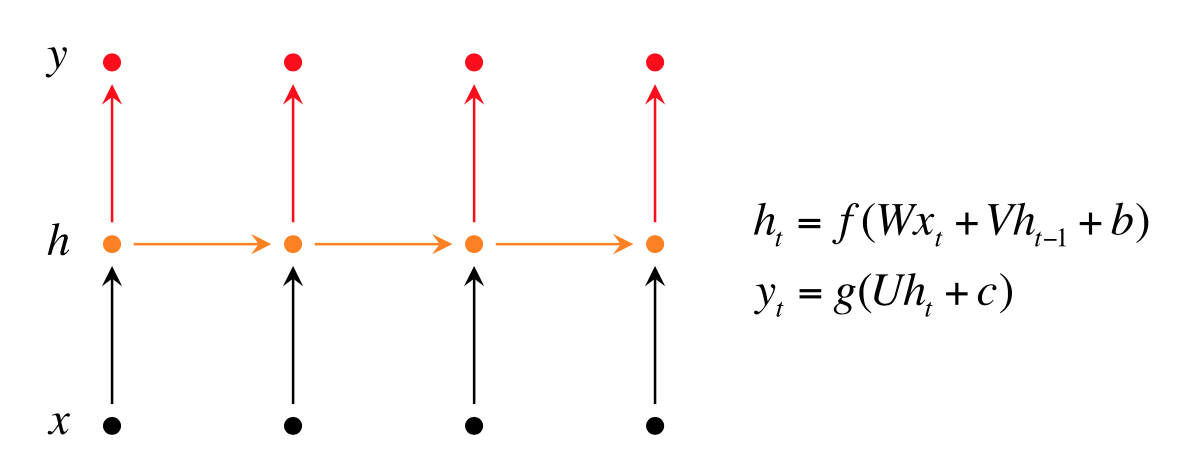### Bidirectional RNNs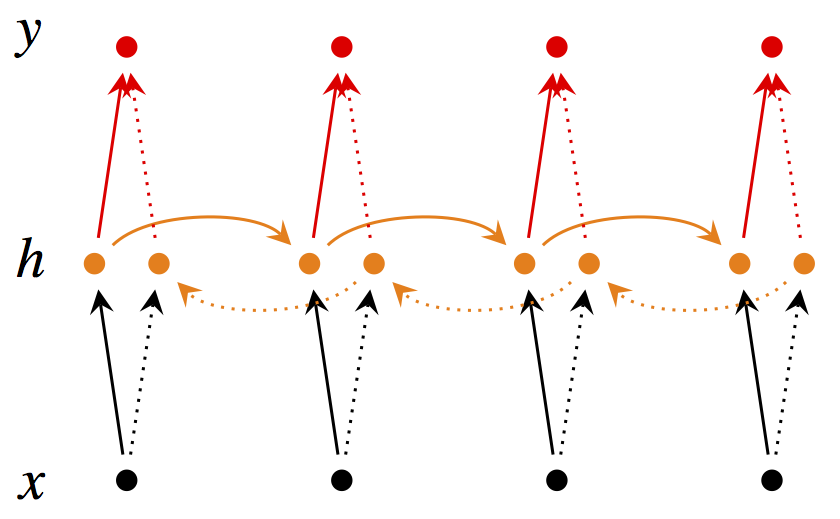$$\begin{equation} \overrightarrow{h}_t = f(\overrightarrow{W} x_t + \overrightarrow{V} \overrightarrow{h}_{t-1} + \overrightarrow{b}) \end{equation}$$

$$\begin{equation} \overleftarrow{h}_t = f(\overleftarrow{W} x_t + \overleftarrow{V} \overleftarrow{h}_{t+1} + \overleftarrow{b}) \end{equation}$$

$$\begin{equation} \hat{y}_t = g(U h_t + c) = g(U [\overrightarrow{h}_t; \overleftarrow{h}_t] + c) \end{equation}$$

### Deep Bidirectional RNNs$$\begin{equation} \overrightarrow{h}_t^{(i)} = f(\overrightarrow{W}^{(i)} h_t^{(i-1)} + \overrightarrow{V}^{(i)} \overrightarrow{h}_{t-1}^{(i)} + \overrightarrow{b}^{(i)}) \end{equation}$$

$$\begin{equation} \overleftarrow{h}_t^{(i)} = f(\overleftarrow{W}^{(i)} h_t^{(i-1)} + \overleftarrow{V}^{(i)} \overleftarrow{h}_{t+1}^{(i)} + \overleftarrow{b}^{(i)}) \end{equation}$$

$$\begin{equation} \hat{y}_t = g(U h_t + c) = g(U [\overrightarrow{h}_t^{(L)}; \overleftarrow{h}_t^{(L)}] + c) \end{equation}$$

### 评测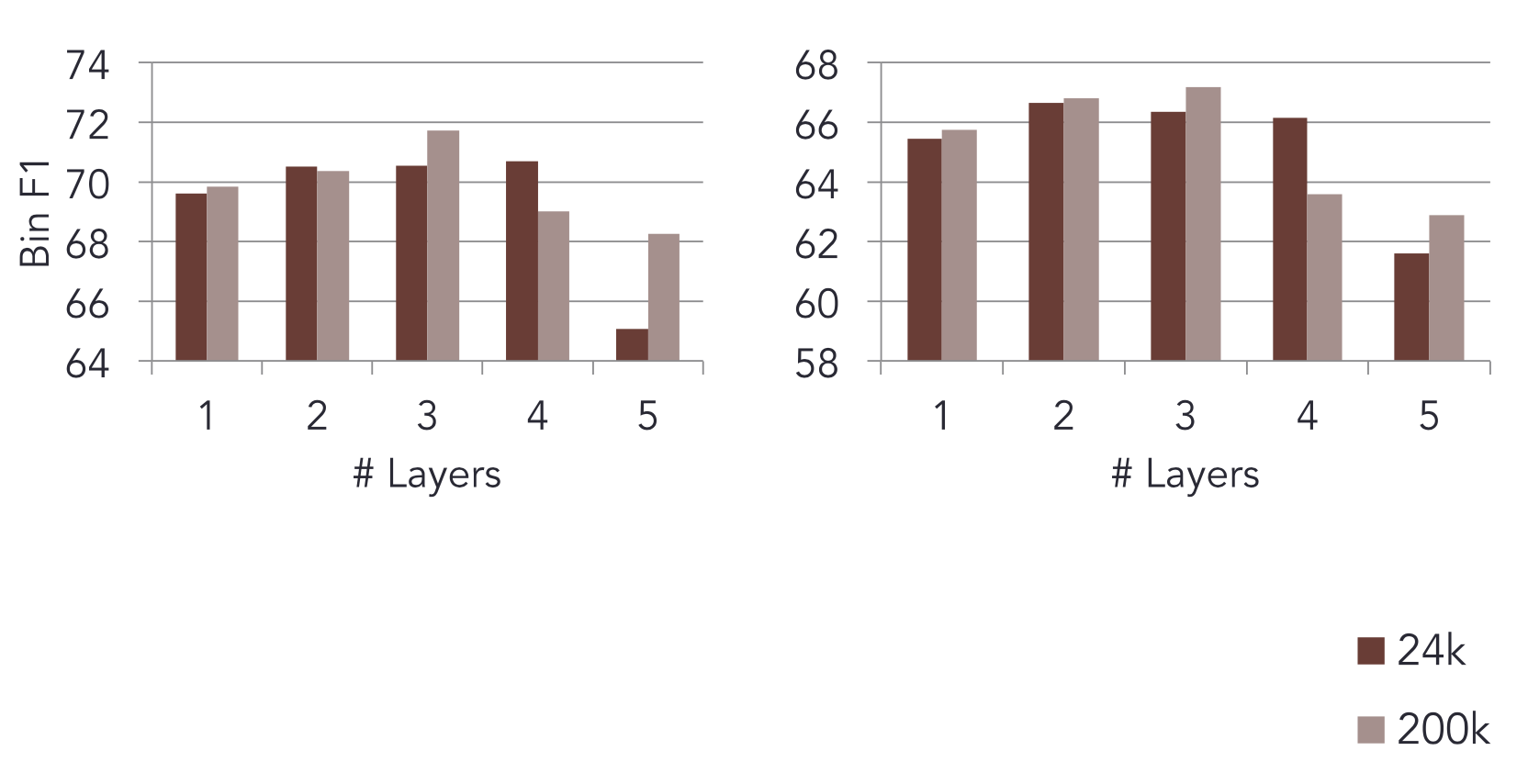### 应用：RNN机器翻译模型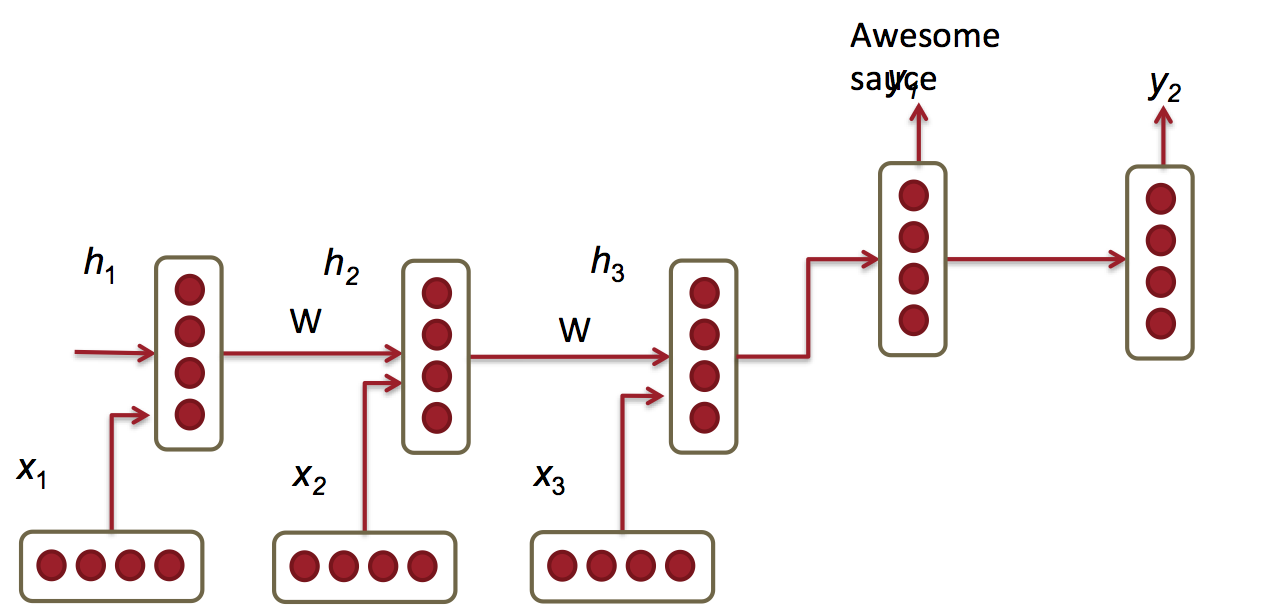$$\begin{equation} h_t = \phi (h_{t-1}, x_t) = f (W^{(hh)} h_{t-1} + W^{(hx)} x_t) \end{equation}$$

$$\begin{equation} h_t = \phi (h_{t-1}) = f (W^{(hh)} h_{t-1}) \end{equation}$$

$$\begin{equation}y_t = softmax (W^{(S)}h_t)\end{equation}$$

$$\begin{equation}\max_{\theta} \dfrac {1}{N} \sum_{n=1}^{N} \log p_{\theta} (y^{(n)}|x^{(n)})\end{equation}$$

1、encoder和decoder使用不同的权值矩阵。也就是上述两个$W^{(hh)}$不再相同。

2、decoder中的隐藏层的输入来自3个方面：

• 前一个时刻的隐藏层

• encoder的最后一个隐藏层($c = h_T$)

• 前一个预测结果 $\hat{y}_{t-1}$

这样导致decoder函数变为：

$$\begin{equation} h_{t} = \phi (h_{t-1}, c, y_{t-1}) \end{equation}$$

•3、使用深度RNN

4、使用 bi-directional encoder

5、不再用$\textit{A B C} \to \textit{X Y}$作为训练实例，而是逆转原文词序：$\textit{C B A} \to \textit{X Y}$。因为A更可能翻译为X，而梯度消失导致A无法影响输出，倒过来A离输出近一些。

## 回顾

• RNN是最好的DeepNLP模型之一

• 因为梯度消失和梯度爆炸，训练很难

• 可以用很多技巧来训练

• 下次课将介绍更强大的RNN拓展：LSTM和GRU

### 评论 5

1. #2

感谢分享知识

littlehann4年前 (2019-05-16)回复
2. #1

谢谢博主！写的很好！就是有一个小问题就是，最后一张图的 c 这个向量我查阅了以下原paper，原话是 is a summary c of the whole input sentence. 是不是这里你写的有误呢

林青城6年前 (2017-08-24)回复
• LSTM最后一个时刻的hidden state就是summary of the whole input sentence

hankcs6年前 (2017-11-23)回复
• 注意是summary 不是sum. 就是最后一个节点的输出.

chao6年前 (2017-12-26)回复